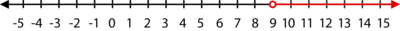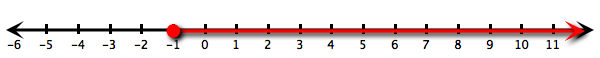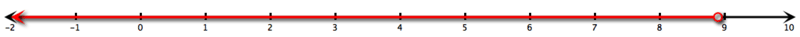# 3.5: Inequalities with Addition and Subtraction

•• Contributed by CK12
• CK12

## Inequalities with Addition and Subtraction

Suppose your favorite baseball team had w wins last year, and this year it vows to win 10 more games. It also promises that the number of its wins this year will be greater than or equal 85. Could you determine how many wins the team had last year?

### Inequalities Using Addition or Subtraction

To solve inequalities, you need the Addition Property of Inequality.

The Addition Property of Inequality states that for all real numbers a, b, and c:

If x<a, then x+b<a+b.

If x<a, then x−c<a−c.

The two properties above are also true for ≤ or ≥.

Because subtraction can also be thought of as “add the opposite,” these properties also work for subtraction situations.

Just like one-step equations, the goal is to isolate the variable, meaning to get the variable alone on one side of the inequality symbol. To do this, you will cancel the operations using inverses.

#### Let's solve the following inequalities:

1. Solve for x: x−3<10.

To isolate the variable x, you must cancel “subtract 3” using its inverse operation, addition.

x−3+3<10+3

x<13

Now, check your answer. Choose a number less than 13 and substitute it into your original inequality. If you choose 0, and substitute it you get:

0−3<10=−3<10

What happens at 13? What happens with numbers greater than 13?

1. Solve for x: x+4>13.

x+4>13

x+4−4>13−4 Subtract 4 from both sides of the inequality.

x>9 Simplify.

The solution is shown below in a graph:1. Solve for x: x+23≥−13.

x+(2/3)≥−(1/3)

x+(2/3)−(2/3)≥−(1/3)−(2/3) Subtract 23 from both sides of the inequality.

x≥−1 Simplify.### Examples

Example 3.5.1

Earlier, you were told that your favorite baseball team had w wins last year and this year it vows to win 10 more games. The team also promises that the number of its wins this year will be greater than or equal to 85. How many wins did the team have last year?

Solution

This year, if the team wins 10 more games than it did last year, it will win w+10 games. They promised that this will be greater than or equal to 85. The inequality representing this situation would then be:

w+10≥85

To solve this, subtract 10 from both sides of the inequality:

w+10−10≥85−10

w≥75

Therefore, the team had at least 75 wins last year.

Example 3.5.2

Solve for y: 5.6>y−3.4.

Solution

Add 3.3 to both sides of the inequality.Simplify.

5.6>y−3.3

5.6+3.3>y−3.3+3.3

8.9>y### Review

Solve each inequality and graph the solution on a number line.

1. x−1>−10
2. x−1≤−5
3. −20+a≥14
4. x+2<7
5. x+8≤−7
6. 5+t≥3/4
7. x−5<35
8. 15+g≥−60
9. x−2≤1
10. x−8>−20
11. 11+q>13
12. x+65<100
13. x−32≤0
14. x+68≥75
15. 16+y≤0

Mixed Review

1. Write an equation containing (3, –6) and (–2, –2).
2. Simplify: |2−11×3|+1.
3. Graph y=−5 on a coordinate plane.
4. y varies directly as x. When x=−1, y=4/5. Find y when x=16/3.
5. Rewrite in slope-intercept form: −2x+7y=63.

### Vocabulary

Term Definition
Addition Property of Inequality For all real numbers a, b, and c: If x<a, then x+b<a+b. If x<a, then x−c<a−c.